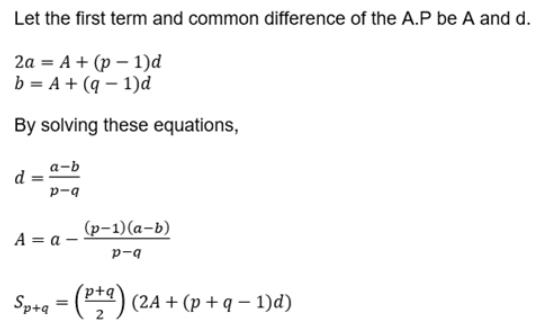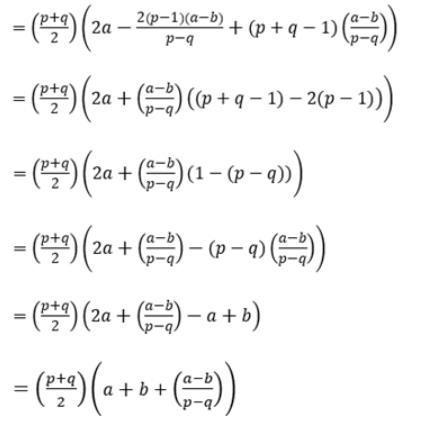Courses

# Test: Sequences And Series (Competition Level) - 1

## 22 Questions MCQ Test Mathematics (Maths) Class 11 | Test: Sequences And Series (Competition Level) - 1

Description
This mock test of Test: Sequences And Series (Competition Level) - 1 for JEE helps you for every JEE entrance exam. This contains 22 Multiple Choice Questions for JEE Test: Sequences And Series (Competition Level) - 1 (mcq) to study with solutions a complete question bank. The solved questions answers in this Test: Sequences And Series (Competition Level) - 1 quiz give you a good mix of easy questions and tough questions. JEE students definitely take this Test: Sequences And Series (Competition Level) - 1 exercise for a better result in the exam. You can find other Test: Sequences And Series (Competition Level) - 1 extra questions, long questions & short questions for JEE on EduRev as well by searching above.
QUESTION: 1

### The first term of an A.P. of consecutive integer is p2 + 1. The sum of (2p + 1) terms of this series can be expressed as

Solution: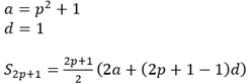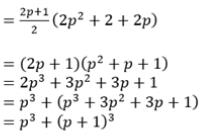QUESTION: 2

Solution:
QUESTION: 3

### If x ∈ R, the numbers 51+x + 51-x, a/2, 25x + 25-x form an A.P. then `a' must lie in the interval;

Solution: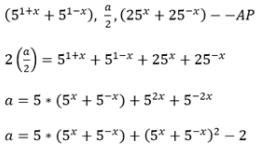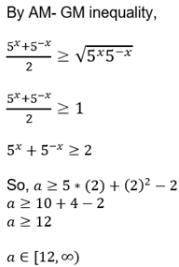QUESTION: 4

The third term of a G.P. is 4. The product of the first five terms is

Solution:
QUESTION: 5

If S is the sum of infinity of a G.P. whose first term is `a', then the sum of the first n terms is

Solution:
QUESTION: 6

The sum of the series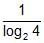+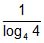+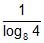+ ....... +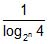is

Solution: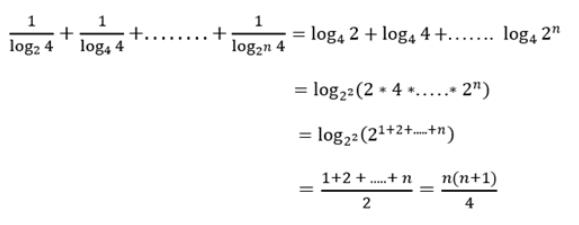QUESTION: 7

For a sequence {an}, a= 2 and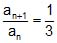. Then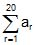is

Solution: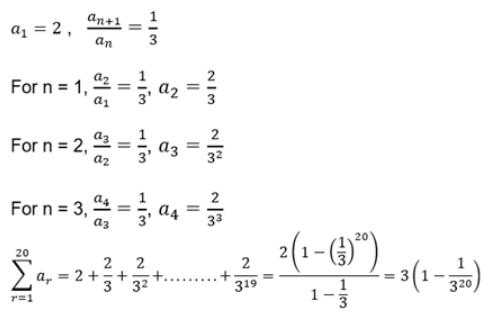QUESTION: 8

a, b be the roots of the equation x2 – 3x + a = 0 and g, d the roots of x2 – 12x + b = 0 and numbers a, b, g, d (in this order) form an increasing G.P., then

Solution: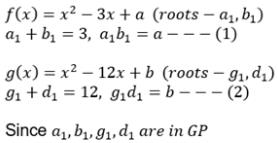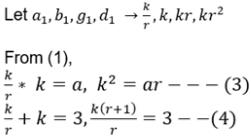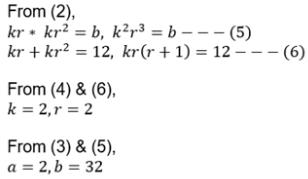QUESTION: 9

If 3 + 1/4 (3 + d) + 1/42 (3 + 2d) + .... + upto ∞ = 8, then the value of d is

Solution: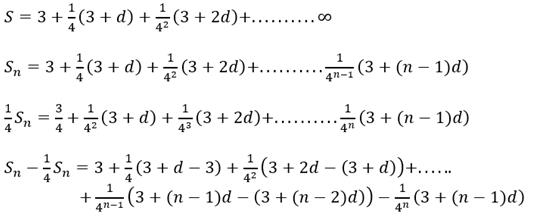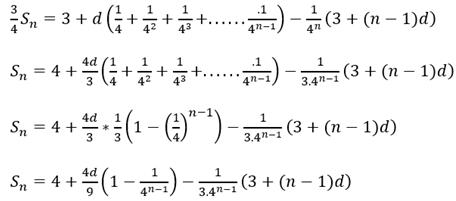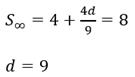QUESTION: 10

The sum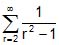is equal to

Solution:QUESTION: 11

In a G.P. of positive terms, any term is equal to the sum of the next two terms. The common ratio of the G.P. is

Solution: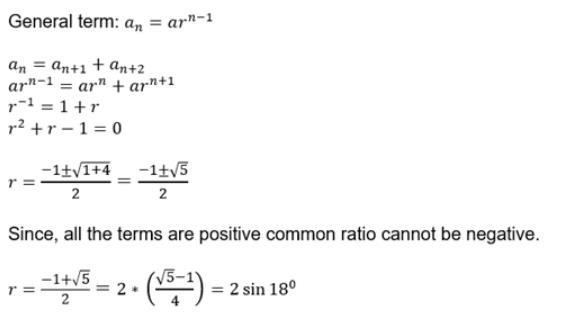QUESTION: 12

If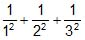+ ...... up to ∞ =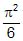, then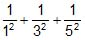+ ..... =

Solution: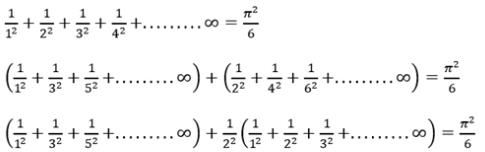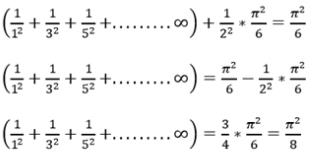QUESTION: 13

Sum of the series S = 12 – 22 + 32 – 42 + ..... – 20022 + 20032 is

Solution:
QUESTION: 14

If x =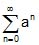, y =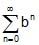, z =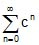where a, b, c are in AP and |a| < 1, |b| < 1, |c| < 1, then x, y, z are in

Solution: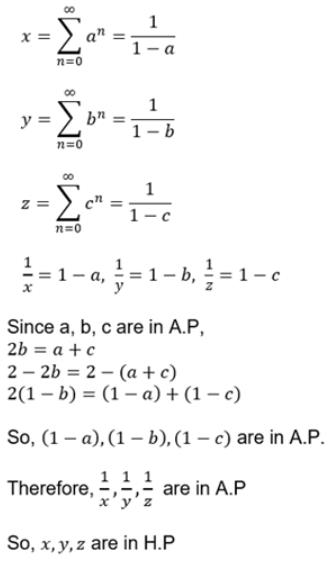QUESTION: 15

The sum to n term of the series 1(1!) + 2(2!) + 3(3!) + ....

Solution: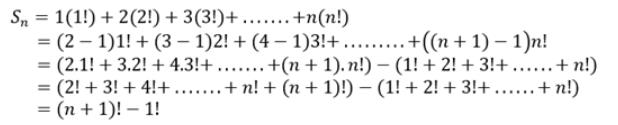QUESTION: 16

The sum to 10 terms of the series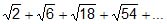is

Solution:
QUESTION: 17

If p is positive, then the sum to infinity of the series,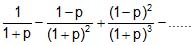is

Solution:
QUESTION: 18

The positive integer n for which 2 × 22 + 3 × 23 + 4 × 24 + ..... + n × 2n = 2n + 10 is

Solution:
QUESTION: 19

If x > 0, and log2 x + log2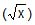+ log2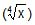+ log2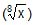+ log2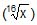+ ....= 4, then x equals

Solution:
QUESTION: 20

If a, b, c are in A.P. p, q, r are in H.P. and ap, bq, cr are in G.P., then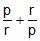is equal to

Solution: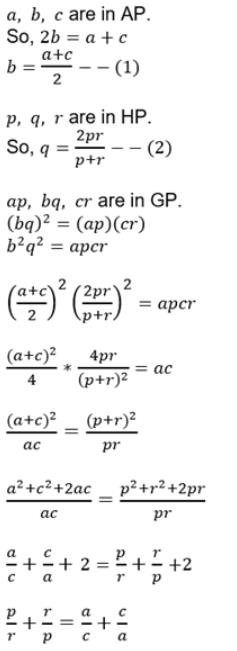QUESTION: 21

12 + 22 + ......+ n2 = 1015, then value of n is

Solution:
QUESTION: 22

If a and b are pth and qth terms of an AP, then the sum of its (p + q) terms is

Solution: